﻿ Determination of the Shape Function of a Multiple Cracked Beam Element and Its Application for the Free Vibration Analysis of a Multiple Cracked Frame Structure

### Determination of the Shape Function of a Multiple Cracked Beam Element and Its Application for the F...

Tran Van Lien, Trinh Anh HaoOPEN ACCESSPEER-REVIEWED

## Determination of the Shape Function of a Multiple Cracked Beam Element and Its Application for the Free Vibration Analysis of a Multiple Cracked Frame Structure

Tran Van Lien1,, Trinh Anh Hao2

1Faculty of Civil Engieering, University of Civil Engineering, Hanoi, Vietnam

2V-CIC, Hanoi, Vietnam

### Abstract

Assessment of the behavior of damaged structures as well as determination of the locations and the depths of cracks in multiple cracked structures are very important and attractive for many researchers. This article presents some results on the determination of the vibration shape function of a multiple cracked elastic beam element, which is modeled as an assembly of intact sub-segments connected by massless rotational springs. Algorithms and computer programs to analyse changes of natural mode shapes of multiple cracked beams have been determined. Numerical analysis of natural mode shapes of cracked simple support beams using the obtained expression shows a good agreement in comparison with the well-known analytical methods. The methodology approach and results presented in this article are new and the basis for building an efficient method to identify cracks in frame structures using wavelet analysis of mode shapes.

### At a glance: Figures

12345
Prev Next

• Lien, Tran Van, and Trinh Anh Hao. "Determination of the Shape Function of a Multiple Cracked Beam Element and Its Application for the Free Vibration Analysis of a Multiple Cracked Frame Structure." American Journal of Civil Engineering and Architecture 2.1 (2014): 12-25.
• Lien, T. V. , & Hao, T. A. (2014). Determination of the Shape Function of a Multiple Cracked Beam Element and Its Application for the Free Vibration Analysis of a Multiple Cracked Frame Structure. American Journal of Civil Engineering and Architecture, 2(1), 12-25.
• Lien, Tran Van, and Trinh Anh Hao. "Determination of the Shape Function of a Multiple Cracked Beam Element and Its Application for the Free Vibration Analysis of a Multiple Cracked Frame Structure." American Journal of Civil Engineering and Architecture 2, no. 1 (2014): 12-25.

 Import into BibTeX Import into EndNote Import into RefMan Import into RefWorks

### 1. Introduction

The formation and development of cracks or damages in construction structures reduces their likelihood of working and lifetime. Therefore, the exact evaluation of the appearance of cracks or damages in the structures is necessary, important issues and attracts the attention of many researchers and civil engineer over the world and in Vietnam.

The current researches on the identification of cracks or damages in structures using non-destructive test method have been developed primarily based on the dynamic characteristics of structures such as natural frequency, mode shapes, response spectrum function [1, 2, 3, 5, 6, 9, 12, 17, 18, 19, 20]. These dynamic characteristics are determined by the analytical method, the semi-analytical method, the finite element method (FEM), and the dynamic stiffness method (DSM).

The analytical and semi-analytical methods are limited in a simple beam [1, 6, 9] and not applicable for complex structures such as multi-span continuous beams or frame structures. Therefore, the identification of dynamic characteristics of structures is based mainly on the FEM and the DSM:

- In the FEM, the multiple-cracked beam element has been modeled as an assembly of intact sub-segments connected by massless rotational springs at the location of cracks or damage. Sato  has developed a combination of the transfer matrix method (TMM) and the FEM for vibration analysis of beam with abrupt changes of cross-section. Gounaris and Dimarogonas  have conjugated the FEM with the idea of a compliance matrix to develop a specific technique for vibration analysis of cracked beam. Zheng and Kessissoglou  has used the overall additional flexibility matrix instead of the local additional flexibility matrix to obtain the total flexibility matrix of a cracked beam. FEM is an approximate method in comparison with the analytical method, especially high natural frequencies and mode shapes [2, 10].

- The idea is to overcome the above-mentioned limitation of FEM and to extend the advantages of the analytical method to more complex structures other than beams in order to develop the DSM. When the FEM is applied for the dynamic problems, only one thing must be estimated which is the field of displacements approximated in the field of static displacement. This means that the dynamic components of the field of displacement are ignored. If we choose the shape function of finite element to be the field of dynamic displacement satisfied by the dynamic equilibrium equations, the FEM is no longer an approximate method, which is an accurate method. For choosing the shape function of dynamic displacement, we must consider movement balance of finite element in the frequency domain, i.e. dynamic displacement with complex amplitude depends on the frequency. After that, the computed steps of the DSM are the same as the FEM. The DSM is created basing on this idea .

In the DSM, multiple cracked structures such as multi-span continuous beams and frames, etc., it is also divided into intact sub-segments joined together at the crack location . Combining the DSM and the TMM with the references of [2, 8], the authors have built straight 3D multiple cracked bar element under axial compression, tension, bending and twisting. The authors have analyzed the change of natural frequencies of structures such as beams, frame-like,… depending of quantities, locations and depths of cracks [2, 18]. The authors have also applied for the determination of the quantities, locations and depths of cracks in the multiple-cracked beam based on natural frequencies measured from experiments [2, 14]. The advantage of these researches is that measured parameters to determine the quantities, location and depth of cracks or damage in the structure may be less than the number of parameters found out on solving extreme value problem. However, mode shapes corresponding to measured natural frequencies have not been found yet.

To determine the mode shapes, it is necessary to find the shape functions for the multiple-cracked beams with arbitrary depths and positions of cracks with an arbitrary coefficient damping. This issue is quite complex and have not been published.

This paper presents some results on the determination of the shape function of a multiple cracked elastic beam subjected to bending, which is modeled as intact sub-segments joined together at the crack positions. Numerical analysis of the shape function of the cracked simple support beams using the obtained expression shows a good agreement in comparison with the well-known analytical methods. A computer program for analysing the changes in mode shapes of a frame structure with multiple-cracked elements is built. The proposed method and the obtained results are new and they are the basis for the determination of the cracks in the structures using wavelet analysis of mode shapes.

### 2. The Shape Function of Intact Lateral Beam

According to FEM as described in , the shape function of intact lateral beam (Figure 1) is the solution of static equilibrium differential equation without external forces as follows: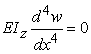(1)

The boundary conditions are: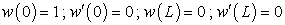(2a)(2b)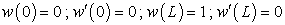(2c)(2d)

The solutions of equation (1) with boundary conditions (2 a-d) are the shape functions N1, N2, N3, N4, called Hermit functions: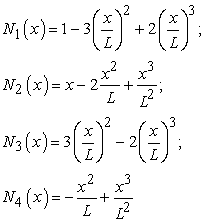(3)

Let the boundary value beat both end nodes, the transverse displacement of the beam at the section x is:(4)

According to DSM developed by Leung , shape functions of the intact beam are the solutions of the undamping free vibration equation:Suppose that, where Φ (x, ω) is the transverse displacement of the beam at the section x, we yield:(5)

The solutions of equation (5) with boundary conditions (2 a-d) are shape functions N1, N2, N3, N4 as follows:(6)

Whereis the dynamic parameter; ω is the circular frequency (rad / s); Fi (i = 1, .., 6) are trigonometric functions as follows: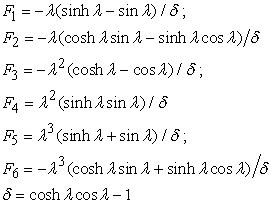When ω=0 corresponding to the static problem. From the shape functions (6) we receive the Hermit shape functions (3).

In the case of intact beam, the determination of shape functions is the first step to obtain the dynamic stiffness matrix of beam subject to bending. In the case of multiple cracked beam, it will be modelled by rotation spring in the position of the crack, the determination of shape function is more complicated problem. In this case, it is necessary to base on the dynamic stiffness matrix obtained by the TMM.

### 3. The Dynamic Stiffness Matrix of a Multiple Cracked Beam

Let us consider a beam of length L subjected to bending on surface Oxy, cross-section area A = b h, moment of inertia I and Young's modulus E, mass density ρ. Free vibration of the beam is described by the following equation :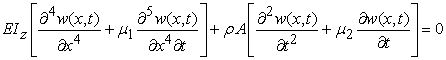Where μ1, μ2 are the material and viscous damping coefficients, respectively. Suppose that, in which Φ (x, ω) is the transverse displacement of the beam at the section x, we yield the equation:(7)

Whereis the dynamic parameter (ω is the circular frequency rad / s). When λ = 0 corresponding to ω = 0 it is a static deformation. When with ω 0 then Φ (x, ω) is a vibration amplitude;is complex modulus.

Suppose that the beam has cracks at positions xj with the depths of aj,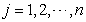, where(Figure 2). The cracks are modeled as rotational springs with the stiffness kzj calculated by converting formulas [15, 16]. In the combination with equation (7), we have the following compatible relations at the crack positions xj: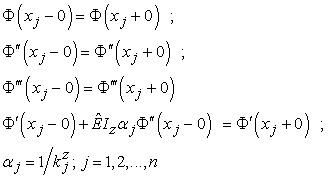(8)

We introduce a vector of nodal displacementsat x = 0 and x = L: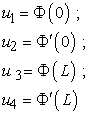and a vector of nodal forces:Also, we introduce the following notations: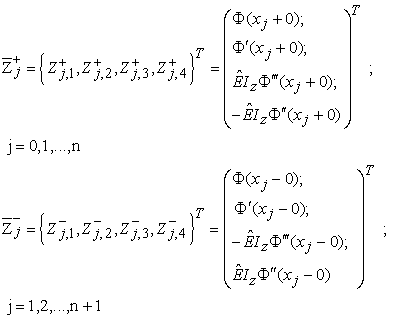then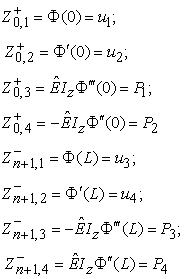and Krylov functions:The general solution of equation (7) for the sub-segment j = 1, 2, ..., n + 1 with x ∈ (xj-1, xj) has the form of:(9)

At right node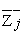of the sub-segment j, we yield:(10)

Where the matrixis called the transfer matrix of uncracked sub-segments beam: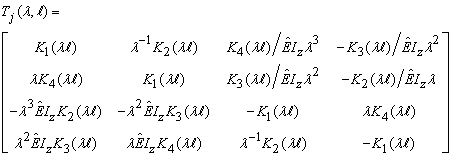with. Using vectors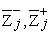, the conditions (8) are rewritten in the matrix form: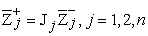(11)

Where the matrixis called the joint transfer matrix for the crack position: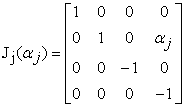Using (11) and (10), we obtained: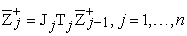(12)

So that: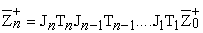Multiplying the both-hand side of the above relation by the matrixand then applying formula (10), we obtain the expression: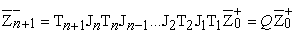(13)

Where the matrix Q is called the transfer matrix for the beam with n cracks: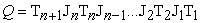For convenience, the matrix Q is rewrite as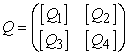, where:Using these notations for the vector of the nodal displacements and vector of the nodal forces given from above, in connection with the expression (13) we obtain the relation between the end forces and the nodal displacements:We rewrite the above equation as follows:(14)

Where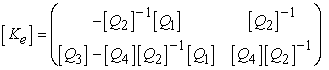(15)

The matrix [Ke]4x4 is called the dynamic stiffness matrix for the multiple cracked beam.

### 4. Determination of the Shape Functions and Mode Shapes of Multiple Cracked Beam Elements

4.1. Shape Functions

Using the general solution (9) and equation (14), we can determine the free vibration shape function of multiple cracked beam elements as following:

a) In order to find the shape function N1, we determine the nodal forces P1 and P2 based on equation (14) with the boundary condition (2 a)The initial parameters of first sub-segment (j = 1) are:Therefore, the shape function N1 for the first sub-segment is:By the same way, using the expression (12) and solution (9), we get the shape function N1 for the next sub-segment. In the case of intact beam, the shape function has the formula:Where. When damping coefficients are zero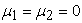, one will get the shape function N1 following the expression (6).

b) To find the shape function N2, we determine the nodal forces P1 and P2 based on equation (14) with the boundary condition (2 b)Also, the initial parameters of the first sub-segment (j = 1) are: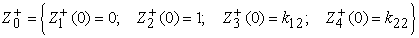Then the shape function N2 for the first sub-segment is:Similarly, using the expressions (12) and solution (9), we get the shape function N2 for the next sub-segment. In the case of intact beam, the shape function has the formula: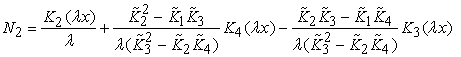When damping coefficients are zero, one will get the shape function N2 following the expression (6).

c) To find the shape function N3, we determine the nodal forces P1 and P2 based on equation (14) with the boundary condition (2 c)The initial parameters of the first sub-segment (j = 1) are:So, the shape function N3 for the first sub-segment is:Using the expressions (12) and solution (9), we get the shape function N3 for the next sub-segment. In case of the intact beam, the shape function has the formula:When damping coefficients are zero, one will get the shape function N3 following the expression (6).

d) To find the shape function N4, we determine the nodal forces P1 and P2 based on equation (14) with the boundary condition (2 d)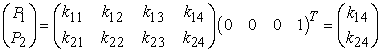The initial parameters of the first sub-segment (j = 1) are: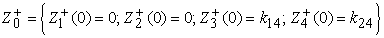The shape function N4 for the first sub-segment is: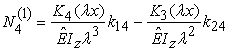Using the expressions (12) and solution (9), we get the shape function N4 for the next sub-segment. In case of the intact beam, the shape function has the formula:When damping coefficients are zero, one will get the shape function N4 following expression (6).

4.2. Determine the Shape Modes

The dynamic stiffness matrices of structure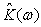are assembled similarly as FEM from the dynamic stiffness matrices [Ke] of each beam elements as presented in equation (15). Thus, the problem of free vibration of structures with the multiple-cracked bar elements leads to the determination of natural frequencies and mode shapes from the following equation: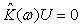(16)

Where the natural frequencies ωj are determined from the equation: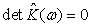(17)

The nodal displacements U corresponding to the natural frequencies ωj will be determined by equations (16). Having obtained the nodal displacements, we receive the mode shapes of the structure with the multiple-cracked beam elements from the expression (4).

### 5. Numerical Results and Discussion

5.1. Simple Support Beam

Let us consider a simple support beam with the following parameters: beam length L = 0.8 m; cross-section area A = b × h = 0.02 × 0.02 (m2); Young’s modulus E = 2.1 × 1011 (N/m2), the Poisson coefficient ν = 0.3 and material mass density ρ = 7800 kg/m3 .

Figure 3 a-b show the changes in fisrt two mode shapes (the diffenrences between the mode shapes obtaned from cracked and uncracked beams) of the simple support beam with one crack at position x=0.3 m from the left node end of the beam and the crack depth of 30% calculated by the analytical method (line ---, [1, 2]) and the proposed method (dash dot line -*-). These figures present a good agreement between the numerical results obtained by the proposed method and the well-known analytical methods.

5.2. Multiple-Span Continuous Beam

Let us consider the multiple-span continuous beam with the following parameters: L1 = 0.8 m, L2 = 1.1 m, L3 = 0.6 m, cross-section area b × h = 0.04 × 0.02 m2, Young’s modulus E = 2.1 × 1011 (N/m2), the Poisson coefficient ν = 0.3 and material mass density ρ = 7800 kg/m3 on Figure 4.

Figure 5, Figure 6, Figure 7 show the changes in the fisrt three mode shapes of the structrure with one crack, the depth of crack ranges from 10% to 60% and the positions of cracks are as followings:

- At 0.2 m (Figure 5 a, Figure 6 a, Figure 7 a), 0.4 m (Figure 5 b, Figure 6 b, Figure 7 b), 0.6 m (Figure 5 c, Figure 6 c, Figure 7 c) from the left node of the first span;

- At 0.2 m (Figure 5 d, Figure 6 d, Figure 7 d), 0.5 m (Figure 5 e, Figure 6 e, Figure 7 e), 0.8 m (Figure 5 f, Figure 6 f, Figure 7 f) from the left node of the second span;

- At 0.1 m (Figure 5 g, Figure 6 g, Figure 7 g), 0.3 m (Figure 5 h, Figure 6 h, Figure 7 h), 0.5 m (Figure 5 i, Figure 6 i, Figure 7 i) from the left node of the third span.

Figure 8. The comparison of the first three mode shapes of multi-span continuous beam with the number of cracks increases from 1 to 6 with equidistance 0.15m on the second span and with invariable crack depth of 30%

Figure 8 shows the changes in the first three mode shapes of multiple cracked multiple-span continuous beam when the number of cracks increases from 1 to 6 with an equidistance 0.15 m on the second span and with invariable crack depth of 30%.

We have the remarks:

a). At crack positions, the diffenrences of the mode shape have bumpy line with the peak on the same crack position, but it is not maximum value (Figure 5 g, Figure 6 c, Figure 6 d, Figure 6 g, Figure 7 c, Figure 7 e, Figure 7 f, Figure 7 g, Figure 7 i).

b). The differences of the mode shapes increase with the increase of the crack depth.

c). At the cracked span, the mode shapes change suddenly, but on the other uncracked spans the mode shapes change smoothly, it is also relative to change large scale on adjacent spans.

d). There are some positions in which the cracks do not influence the mode shapes, for example: the crack at position of 0.2 m causes the change in the first two mode shapes but does not cause the change in the third mode shape (Figure 7 a). Thus, these positions of the cracks are called the invariable point of mode shape to discriminate between them with the fixed position in which the mode shapes have a zero amplitude value.

e). When gradually increasing the number of cracks with equidistance, then the change amplitude of the mode shape also increases but the magnitude are not necessarily the largest (Figure 8).

5.3. 2D Frame

Let us consider the frame of two stories and one span with the following parameters: length span L = 2.4 m, height storey H = 2.8 m, cross-section area b × h = 0.14 × 0.24 m2, Young’s modulus E = 3.5 × 1010 (N/m2), the Poisson coefficient ν = 0.3 and material mass density ρ = 2500 kg/m3 (Figure 9 a). The axial deformation of column is ignored.

Figure 9 b-c show the first three mode shapes of the two-storey frame without crack. The obtained shapes are quite similar to those of previous author's , which is asymmetric on the first and second mode shapes and is symmetric on the third mode shape.

Figure 10. The comparison of the first mode shape of the frame with the crack depth ranging from 10%-60% and variable position on frame elements
Figure 11. The comparison of the second mode shape of the frame with the crack depth ranging from 10%-60% and variable position on frame elements
Figure 12. The comparison of the third mode shape of the frame with the crack depth ranging from 10% - 60% and variable position on frame elements

Figure 10, Figure 11 and Figure 12 show the changes in the first three mode shapes of the frame with one crack, in which the depth of crack ranges from 10% to 60% and the position of cracks are as following:

- For column 1: 0.6 m (Figure 10 a, Figure 11 a, Figure 12 a), 1.4 m (Figure 10 b, Figure 11 b, Figure 12 b), 2.2 m (Figure 10 c, Figure 11 c, Figure 12 c).

- For beam 3: 0.4 m (Figure 10 d, Figure 11 d, Figure12 d), 0.8 m (Figure 10 e, Figure 11 e, Figure 12 e), 1.2 m (Figure 10 f, Figure 11 f, Figure 12 f).

- For column 4: 0.6 m (Figure 10 g, Figure 11 g, Figure 12 g), 1.4 m (Figure 10 h, Figure 11 h, Figure 12 h), 2.2 m (Figure 10 i, Figure 11 i, Figure 12 i).

Figure 13. The comparison of the first three mode shapes of the frame with the invariable crack depth of 30% when the number of cracks on one element increases from 1 to 6

Figure 13 shows the changes in the first three mode shapes of the frame with the invariable crack depth of 30% when the number of cracks on one element increases from 1 to 6 at the following position:

- For column 1: 0.4 m, 0.8 m, 1.2 m, 1.6 m, 2.0 m, 2.4 m (Figure 13 a-c).

- For beam 3: 0.2 m, 0.6 m, 1.0 m, 1.4 m, 1.8 m, 2.2 m (Figure 13 d-f).

- For column 4: 0.4 m, 0.8 m, 1.2 m, 1.6 m, 2.0 m, 2.4 m (Figure 13 g-i).

- For beam 6: 0.2 m, 0.6 m, 1.0 m, 1.4 m, 1.8 m, 2.2 m (Figure 13 j-l).

Figure 14. The comparison of the first three mode shapes of the frame with the invariable crack depth of 30% when the number of cracks on two different elements increases from 1 to 6

Figure 14 shows the changes in the first three mode shapes of the frame with the invariable crack depth of 30% when the number of cracks on two different elements increases from 1 to 6 at the following position:

- For beams 3 and 6: at a distance of 0.3 m from the left node of the beam, increasing equidistant is 0.36 m (Figure 14 a-c);

- For columns 1 and 4: at a distance of 0.4 m from the lower node of the column, increasing equidistant is 0.4 m (Figure 14 d-f);

- For columns 1 and 5: at a distance of 0.4 m from the lower node of the column, increasing equidistant is 0.4 m (Figure 14 g-i);

- For column 1: at a distance of 0.4 m from the lower node of the column, increasing equidistant is 0.4 m. For beam 3: at a distance of 0.4 m from the left node of the beam, increasing equidistant is 0.3 m (Figure 14 j-l);

Along with the remarks similar to multiple-span continuous beam (5.2 a-e), we have additional remarks:

a) Each mode shape is influenced differently by the position of the crack: the crack, which occurs on the position closed to the clamp or joint node, creates more changes on the first two mode shapes than the crack, which occurs on the middle between of element (Figure 10 a, c-d, g, i, Figure 11 a, c-d, g, i). However, the crack, which occurs on the middle between of element, creates more changes on the third mode shape (Figure 12 b, f, h).

b) The crack occurred on the symmetric position (at middle of beam 3) does not create changes in the asymmetric mode shape (Figure 10 f, Figure 11 f), but it creates a strongly change in the symmetric mode shape (Figure 12 f).

c) When gradually increasing the number of cracks with equidistance, then the change amplitude of the mode shape also increases but the magnitude are not necessarily the largest (Figure 13 d-e, k, Figure 14 a-c, j).

### 6. Conclusion

The article presents some results on the determination of the vibration shape functions of a multiple cracked elastic beam element, where the multiple cracked beam element is modeled as an assembly of intact sub-segments connected by massless rotational springs. Also, algorithms and computer programs are established for analysing the changes in natural mode shapes of multiple cracked continuous beams. Numerical analysis of the shape modes of the cracked simple support beams using the proposed method shows a good agreement in comparison with the well-known analytic methods. Details in the changes of mode shapes for free vibration of structure framing system with cracked elements are given. The results received are new, realiabilty and can be used as a basis for building an efficient method to identify cracks in the system framing structure using wavelet analysis of mode shapes.

### References

  Tran Thanh Hai (2011), Diagnosis of cracked beams by using vibration methods, PhD thesis, Institute of Mechanics, Vietnamese.In article  Tran Van Lien (2003), The inverse problems in mechanics and its application, PhD thesis, National University of Civil Engineering, Vietnamese.In article  Adams R.D., Cawley P., Pie C.J. and Stone B.J.A. (1978), “A vibration technique for non-destructively assessing the integrity of structures”, Journal of Mechanical Engineering Science, 20, 93-100.In article CrossRef  Bathe K.J. (1996), Finite Element Procedures, Prence-Hall.In article  Cawley P., Adams R.D. (1979), “The location of defects in structures from measurements of natural frequencies”, Journal of strain analysis, 14 (2).In article  Charti M., Rand R. and Mukherjee S. (1997), “Modal analysis of cracked beam”, Journal of Sound and Vibration, 207, 249-270.In article CrossRef  Nguyen Xuan Hung (1999), Dynamics of structures and its application in structural identification, Institute of Applied Mechanics, National Center for Natural Science and Technology.In article  Khiem N.T., Lien T.V. (2001), “A simplified method for frequency analysis of multiple cracked beam”, Journal of Sound and Vibration, 245 (4), 737-751.In article CrossRef  Nguyen Tien Khiem and Dao Nhu Mai (1997), “Natural frequency analysis of cracked beam”, Vietnam Journal of Mechanics, NCNST of Vietnam, 19 (2), 28-38.In article  Leung A.Y.T. (1993), Dynamic Stiffness and Substructures, Springer-Verlag, London.In article CrossRef  Rao S.S. (1986), Mechanical vibrations. Second Edition, Addison-Wesley Pub Company.In article  Sato H. (1983), “Free vibration of beams with abrupt changes of cross-section”, Journal of Sound and Vibration, 89, 59-64.In article CrossRef  Shrifin E.I. and Ruotolo R. (1999) “Natural frequencies of a beam with an arbitrary number of cracks”, Journal of Sound and Vibration, 222 (3), 409-423.In article CrossRef  Khiem N.T., Lien T.V. (2004), “Multi-crack detection for beam by the natural frequencies”, Journal of Sound and Vibration, 273 (1-2), 175-184.In article CrossRef  Chondros T.G., Dimarogonas A.D. and Yao J. (1998), “A continuous cracked beam vibration theory”, Journal of Sound and Vibration, 215 (1), 17-34.In article CrossRef  Haisty B.S. and Springer W.T. (1988), “A general beam element for use in damage assessment of complex structures”, Journal of Vibration, Acoustics, Stress and Reliability in Design, 110, 389-394.In article CrossRef  Gounaris G., Dimarogonas A. (1988), “A finite element of a cracked prismatic beam for structural analysis”, Computers and Structures, 28, 309-313.In article CrossRef  Khiem N.T., Lien T.V. (2002), “The dynamic stiffness matrix method in forced vibration analysis of multiple cracked beam”, Journal of Sound and Vibration, 254 (3), 541-555.In article CrossRef  Zheng D.Y., Kessissoglou N.J. (2004), “Free vibration analysis of a cracked beam by finite element method”, Journal of Sound and Vibration, Vol 273 (3), 457-475.In article CrossRef  Baris Binici (2005),”Vibration of beam with multiple open cracks subjected to axial force”, Journal of Sound and Vibration, Vol 287 (1-2), 277-295.In article CrossRef  Brasiliano A., Doz G. N., Brito J.L.V. (2004),”Damage identification in continuous beams and frame structures using the residual error method in the movement equation”, Nuclear Engineering and Design, 227, 1-17.In article CrossRef